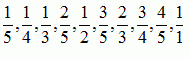Problems

# Farey sequences

Time limit 1 second
Memory limit 128 MiB

A fraction h / k is called a proper fraction if it lies between 0 and 1 and if h and k have no common factors. For any positive integer n1, the Farey sequence of order n, F[n], is the sequence of all proper fractions with denominators which do not exceed n together with the "fraction" 1 / 1, arranged in increasing order. So, for example, F is the sequence:For a given n, you are to find the k-th fraction in the sequence F[n].

## Input data

Input consists of a sequence of lines containing two natural numbers n and k, 1n1000 and k sufficiently small such that there is the k-th term in F[n]. (The length of F[n] is approximately 0.3039635n^2).

## Output data

For each line of input print one line giving the k-th element of F[n] in the format as shown in example.

## Examples

Input example #1
5 5
5 1
5 9
5 10
117 348
288 10000

Output example #1
1/2
1/5
4/5
1/1
9/109
78/197